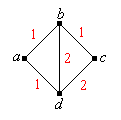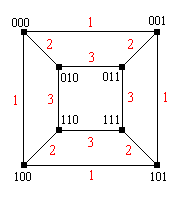]> Random Walks on Graphs

## 12. Random Walks on Graphs

#### Introduction

Suppose that $G S L$ is a connected graph with vertex set $S$ and edge set $L S 2$. We assume that the graph is undirected (perhaps a better term would be bi-directed) in the sense that $x y L$ if and only if $y x L$. We also assume that the graph has no loops, so that $x x L$ for any $x S$. The vertex set $S$ may by infinite. Let $N x y S x y L$ denote the set of neighbors of a vertex $x$, and let $d x N x$ denote the degree of $x$.

Suppose now that there is a conductance $c x y 0$ associated with each edge $x y L$. The conductance is symmetric in the sense that $c x y c y x$ for $x y L$. We extend $c$ to a function on all of $S S$ by defining $c x y 0$ for $x y L$. As the terminology suggests, we imagine a fluid of some sort flowing through the edges of the graph, so that the conductance of an edge measures the capacity of the edge in some sense. The best interpretation is that the graph is an electrical network and the edges are resistors. In this interpretation, the conductance of a resistor is the reciprocal of the resistance.

Let $C x y y S c x y$ and suppose that $C x$ for each $x S$. The Markov chain with state space $S$ and transition probability matrix $P$ given by

$P x y c x y C x , x y S 2$

is called a random walk on the graph $G$. This chain governs a particle moving along the vertices of $G$. If the particle is at vertex $x$ at a given time, then the particle will be at a neighbor of $x$ at the next time; the neighbor is chosen randomly, in proportion to the conductance. In the setting of an electrical network, it is natural to interpret the particle as an electron.

Note that multiplying the conductance function $c$ by a positive constant has no effect on the associated random walk.

Suppose that each edge has the same conductance, so that $c$ is constant on the edges.

1. Show that $C x c d x$ for every $x S$.
2. Thus, note that the basic assumption on $C$ requires that each vertex have finite degree. That is, the graph $G$ must be locally finite.
3. Show that the transition probability matrix is given by $P x y 1 d x y N x 0 y N x$

The chain in the previous exercise is the symmetric random walk on $G$. Thus, if the chain is in state $x$ at a given time, then the chain is equally likely to move to any of the neighbors of $x$ at the next time.

Consider the random walk on the graph below with the given conductance values. The underlying graph is sometimes called the Wheatstone bridge in honor of Charles Wheatstone.1. Explicitly given the transition probability matrix $P$.
2. Suppose that the chain starts at $a$. Find the probability density function of $X 2$

Consider the random walk on the cube graph shown below, with the given conductance values. The vertices are bit strings of length 3, and two vertices are connected by an edge if and only if the bit strings differ by a single bit.1. Explicitly given the transition probability matrix $P$.
2. Suppose that the initial distribution is the uniform distribution on $000 001 101 100$. Find the probability density function of $X 2$.

Note each of the following:

1. If $G$ is connected then $X$ is irreducible.
2. If $G$ is connected and finite then $X$ is irreducible and recurrent.
3. More generally, if $G$ is not connected then the equivalence classes of $X$ are the components of $G$ (the maximal connected subsets of $S$). The finite components are recurrent..

Suppose that $G$ is connected and finite. Show that $X$ is either aperiodic or has period 2. Moreover, $X$ has period 2 if and only if $G$ is bipartite. That is, the vertex set $S$ can be partitioned into sets $A$ and $B$ such that every edge in $L$ has one endpoint in $A$ and one endpoint in $B$; these sets are then the cyclic classes of the chain.

#### Random Walks on $k$

Random walks on the integer lattices $k$, where $k$, are particulalry interesting. Consider first the simple random walk on , with transition probabilities

$P x x 1 p , P x x 1 1 p , x$

where $0 p 1$. Note that the chain is irreducible.

Note that starting in state 0, the chain can return to state 0 only at even times. Moreover, the chain returns to 0 whenever the number of steps right equals the number of steps left. Thus, show that

$P 2 n 0 0 2 n n p n 1 p n , n$

Use Stirling's approximation to show that $P 2 n 0 0 4 p 1 p n n as n$. Conclude that

1. $G 0 0$ and hence the chain is transient if $p 12$.
2. $G 0 0$ and hence the chain is recurrent if $p 12$.

More generally, consider $k$, where $k$ is a positive integer. For $i 1 2 k$, let $u i k$ denote the unit vector with 1 in position $i$ and 0 elsewhere. The simple random walk on $k$ has transition probabilities

$P x x u i p i , P x x u i q i , x k , i 1 2 k$

where $p i$ and $q i$ are positive for each $i$ and $i 1 k p i q i 1$. Clearly, the larger the dimension $k$, the less likely the chain is to be recurrent. In turns out that the behavior when $k 2$ is similar to the behavior when $k 1$: the chain is recurrent only in the symmetric case $p i q i 14$ for each $i$. When the dimension $k$ is 3 or more, the chain is transient for all values of the parameters, even in the symmetric case. Thus, for the simple, symmetric random walk on the integer lattice $k$, we have the following interesting dimensional phase shift: the chain is recurrent in dimensions 1 and 2 and transient in dimensions 3 or more. The proofs are similar in to the proof sketched in the previous two exercises for dimension 1, but the details are considerably more complex. A return to $0$ can occur only at even times and in the symmetric case,

$P 2 n 0 0 C k n k 2 as n$

where $C k$ is a positive constant that depends on the dimension $k$. Thus $G 0 0$ and the chain is recurrent if $k 1 2$; $G 0 0$ and the chain is transient if $k 3 4$.

#### Positive Recurrence and Limiting Distributions

We return now to the general case of a random walk on a graph $G$.

Show that the function $C$ is left-invariant for $P$. Thus, conclude that the random walk is positive recurrent if and only if

$K x S C x x y S 2 c x y$

in which case the invariant probability density function $f$ is given by $f x C x K$ for $x S$.

Consider the special case of the simple random walk on $G$, so that the conductance function $c$ is constant on the set of edges $L$. Show that the random walk is positive recurrent if and only if the set of vertices $S$ is finite, in which case the invariant probability density function $f$ is given by $f x d x 2 m$ for $x S$ where $d$ is the degree function and where $m$ is the number of undirected edges.

Recall, for example, the simple random walk on $k$. We showed that the walk is recurrent if the dimension $k$ is 1 or 2, and so we now know that the walk is null recurrent in these cases. The walk is transient if $k 3$.

Consider the random walk on the Wheatstone bridge given in Exercise 2.

1. Show that the chain is aperiodic.
2. Find the invariant probability density function.
3. Find the mean return time to each state.
4. Find the limit of $P n$ as $n$.

Consider the random walk on the cube graph given in Exercise 3.

1. Show that the chain has period 2 and find the cyclic classes.
2. Find the invariant probability density function.
3. Find the mean return time to each state.
4. Find the limit of $P 2 n$ as $n$.
5. Find the limit of $P 2 n 1$ as $n$.

#### Reversibility

Essentially, all reversible Markov chains can be interpreted as random walks on graphs. This fact is one of the reasons for studying such walks.

Show that a positive recurrent random walk $X$ on a graph $G$ is reversible.

Conversely, suppose that $X$ is a reversible Markov chain on $S$ with transition probability matrix $P$ and invariant probability density function $f$. Suppose also that $P x x 0$ for every $x S$. Show that $X$ can be interpreted as the random walk on the state graph $G$ of $X$ with conductance function $c$ given by

$c x y f x P x y , x y S 2$

Recall that the Ehrenfest chain is reversible. Interpreting the chain as a random walk on a graph, sketch the graph and find a conductance function.